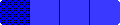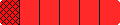Home
 Suggestions for Using Games in Grades 1-2 These first grade students are placing Fraction Bars on the Small Step mat to determine how far they can move their markers.The following sequence of events involves the introduction of Fraction Bars to a second grade class, and illustrates one approach to having students gradually use all the different types of bars. This approach was used to introduce the Small Step Race, but could be used to introduce other games from the Grades 1-2 Teacher' Guide. After using Fraction Bars for two semesters with undergraduates, I received a call from Elsie McDonald, a grade school teacher, asking if I knew of ways to introduce fractions to her second graders. For one semester I met weekly with her second grade students, and this lead to the creation of games and activities for teaching fractions. The first game that was developed was Small Step Race. This game has five orange bars, each divided into 12 equal parts. During the first visit to the school, the second graders played the game by randomly selecting only orange Fraction Bars. As each orange bar was selected, the amount a student's marker could be moved on the race track was determined by counting the number of shaded parts in the bar or by merely placing the bar on the mat. During my second visit to the school, the students played Small Step Race using both the orange bars and the red bars (6 equal parts per bar). They quickly learned that each shaded part of a red bar equaled 2 steps on the race mat. During subsequent visits, blue, yellow and green bars were introduced: each part of a blue bar equals 3 steps on the mat; each part of a yellow bar equals 4 steps; and each part of a green bar equals 6 steps. Students could place the bar they selected on the race mat to see how many steps they could take, but soon they became familiar with the number of steps for eaxh color. For example, each part of a red bar was worth two steps; each part of a blue bar was worth 3 steps; etc.This introduction to Fraction Bars was nonthreatening and all the second graders were successful at playing this game. They grew fond of whole bars (12 steps on the mat) and disliked getting zero bars. In addition to this game, which the students played in groups of 2 to 4, there were class activities and discussions in which groups of students answered questions by using their decks of bars: "How many steps can you move on the mat for a yellow bar with one shaded part?" (Ans. 4) "What are some other bars for which you can take 4 steps on the mat?" (Ans. a red bar with 2 parts shaded or an orange bar with 4 parts shaded. Even before using fraction numerals, the background was being prepared for the part-to-whole concept of a fraction and equality and inequality of fractions.
HomeHome